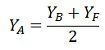# Lap & Wave Winding

The armature winding is the most important part of the rotating machine. It is the place where energy conversion takes place, i.e., the mechanical energy is converted into electrical energy, and the electrical energy is converted into mechanical energy. The armature winding is mainly classified into types, i.e., the lap winding and the wave winding.

## Lap Winding

In lap winding, the conductors are joined in such a way that their parallel paths and poles are equal in number. The end of each armature coil is connected to the adjacent segment on the commutator. The number of brushes in the lap winding is equal to the number of parallel paths, and these brushes are equally divided into negative and positive polarity.The lap winding is mainly used in low voltage, high current machine applications. They are three types

1. Simplex Lap Winding
2. Duplex Lap Winding
3. Triplex Lap Winding

1. Simplex Lap Winding: In simplex lap winding, the terminating end of one coil is joined to the commutator segment and the starting end of the next coil is placed under the same pole. Also, the number of parallel paths is similar to the number of poles of the windings.2. Duplex Winding: In duplex winding the number of parallel paths between the pole is twice the number of poles. The duplex lap winding is mainly used for heavy current applications. Such type of winding is obtained by placing the two similar winding on the same armature and connecting the even number commutator bars to one winding and the odd number to the second winding.3. Triplex Lap Winding: In triplex lap winding the windings are connected to the one-third of the commutator bars.

The lap winding has many paths and hence it is used for the larger current applications. The only disadvantage of the lap winding is that it requires many conductors which increase the cost of the winding.

## Wave Winding

In wave winding, only two parallel paths are provided between the positive and negative brushes. The finishing end of the one armature coil is connected to the starting end of the other armature coil commutator segment at some distance apart.In this winding, the conductors are connected to two parallel paths irrespective of the number of poles of the machine. The number of brushes is equal to the number of parallel paths. The wave winding is mainly used in high voltage, low current machines.

If after passing one round, the armature winding falls into a slot to the left of its initial point, then the winding is said to be retrogressive.And if the armature windings fall on one slot to the right then it is called progressive winding.Assume the two layers winding and suppose that the conductor AB must be at the upper layer half of the slot on the left or right. Consider that the YB is the back pitch and YF is the front pitch. The sum of the back pitch and the front pitch is nearly equal to the pole pitch of the winding.The equation gives average pitch of the windingIf ZA is the total number of conductor or coil side, then the average pitch is expressed by the equation,Where, P – number of poles
Since P is always even, then Z = PY± 2, will always be considered as an even integer.

For progressive winding plus sign will use and for retrogressive winding negative winding will be used.